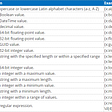# Delete Blank Cells in Excel in C# and VB.NET

• Create an instance of Workbook class.
• Get the worksheet that contains blank cells using Workbook.Worksheets[sheetIndex] property.
• Get the row count and column count using Worksheet.Rows.Count() and Worksheet.Columns.Count() methods.
• Loop through the rows and columns, access the current cell using Worksheet.Range[rowIndex, columnIndex] property. Note the row index and column index here are 1-based.
• Determine if the current cell is blank or not using XlsRange.IsBlank Property. If any blank cell is found, remove it using XlsWorksheet.DeleteRange(CellRange, DeleteOption) method.
• Save the result file using Workbook.SaveToFile() method.
`using Spire.Xls;using System.Linq; namespace DeleteBlankCells{    class Program    {        static void Main(string[] args)        {            //Instantiate a Workbook object            Workbook workbook = new Workbook();            //Load the Excel file            workbook.LoadFromFile("Sample1.xlsx");             //Get the first worksheet            Worksheet sheet = workbook.Worksheets;             //Get the row count            int maxRow = sheet.Rows.Count();            //Get the column count            int maxColumn = sheet.Columns.Count();             //Loop through the rows and columns            for (int i = maxRow - 1; i >= 0; i--)            {                for (int j = maxColumn - 1; j >= 0; j--)                {                    //Get the current cell                    CellRange cell = sheet.Range[i + 1, j + 1];                    //Determine if the cell is blank or not                    if (cell.IsBlank)                    {                        //Remove the blank cell with DeleteOption (MoveLeft/MoveUp)                        sheet.DeleteRange(cell, DeleteOption.MoveLeft);                    }                }            }             //Save the result file            workbook.SaveToFile("DeleteBlankCells.xlsx", ExcelVersion.Version2013);        }    }}`
`Imports Spire.Xls Namespace DeleteBlankCells    Friend Class Program        Private Shared Sub Main(ByVal args As String())            'Instantiate a Workbook object            Dim workbook As Workbook = New Workbook()            'Load the Excel file            workbook.LoadFromFile("Sample1.xlsx")             'Get the first worksheet            Dim sheet As Worksheet = workbook.Worksheets(0)             'Get the row count            Dim maxRow As Integer = sheet.Rows.Count()            'Get the column count            Dim maxColumn As Integer = sheet.Columns.Count()             'Loop through the rows and columns            For i = maxRow - 1 To 0 Step -1                 For j = maxColumn - 1 To 0 Step -1                    'Get the current cell                    Dim cell As CellRange = sheet.Range(i + 1, j + 1)                    'Determine if the cell is blank or not                    If cell.IsBlank Then                        'Remove the blank cell with DeleteOption (MoveLeft/MoveUp)                        sheet.DeleteRange(cell, DeleteOption.MoveLeft)                    End If                Next            Next             'Save the result file            workbook.SaveToFile("DeleteBlankCells.xlsx", ExcelVersion.Version2013)        End Sub    End ClassEnd Namespace`

# Delete Blank Rows in Excel in C# and VB.NET

• Create an instance of Workbook class.
• Get the worksheet that contains blank rows using Workbook.Worksheets[sheetIndex] property.
• Get the row count using Worksheet.Rows.Count() method.
• Loop through the rows in the worksheet.
• Determine whether the current row is blank or not using Worksheet.Rows[rowIndex].IsBlank property. If any blank row is found, remove it using XlsWorksheet.DeleteRow(rowIndex) method (note that the row index in the DeleteRow method starts from 1).
• Save the result file using Workbook.SaveToFile() method.
`using Spire.Xls;using System.Linq; namespace DeleteBlankRows{    class Program    {        static void Main(string[] args)        {            //Instantiate a Workbook object            Workbook workbook = new Workbook();            //Load the Excel file            workbook.LoadFromFile("Sample2.xlsx");             //Get the first worksheet            Worksheet sheet = workbook.Worksheets;            //Get the row count            int rowCount = sheet.Rows.Count();             //Loop through the rows             for (int i = rowCount - 1; i >= 0; i--)            {                //Determine if the row is blank or not                if (sheet.Rows[i].IsBlank)                {                    //Delete blank row                    sheet.DeleteRow(i + 1); //Index parameter in DeleteRow method starts from 1                }            }              //Save the result file            workbook.SaveToFile("DeleteBlankRows.xlsx", ExcelVersion.Version2013);        }    }}`
`Imports Spire.Xls Namespace DeleteBlankRows    Friend Class Program        Private Shared Sub Main(ByVal args As String())            'Instantiate a Workbook object            Dim workbook As Workbook = New Workbook()            'Load the Excel file            workbook.LoadFromFile("Sample2.xlsx")             'Get the first worksheet            Dim sheet As Worksheet = workbook.Worksheets(0)            'Get the row count            Dim rowCount As Integer = sheet.Rows.Count()             'Loop through the rows             For i = rowCount - 1 To 0 Step -1                'Determine if the row is blank or not                If sheet.Rows(i).IsBlank Then                    'Delete blank row                    sheet.DeleteRow(i + 1) 'Index parameter in DeleteRow method starts from 1                End If            Next             'Save the result file            workbook.SaveToFile("DeleteBlankRows.xlsx", ExcelVersion.Version2013)        End Sub    End ClassEnd Namespace`

# Delete Blank Columns in Excel in C# and VB.NET

`using Spire.Xls;using System.Linq; namespace DeleteBlankRowsAndColumns{    class Program    {        static void Main(string[] args)        {            //Instantiate a Workbook object            Workbook workbook = new Workbook();            //Load the Excel file            workbook.LoadFromFile("Sample3.xlsx");             //Get the first worksheet            Worksheet sheet = workbook.Worksheets;            //Get the column count            int columnCount = sheet.Columns.Count();             //Loop through the columns             for (int j = columnCount - 1; j >= 0; j--)            {                //Determine if the column is blank or not                if (sheet.Columns[j].IsBlank)                {                    //Delete blank column                    sheet.DeleteColumn(j + 1); //Index parameter in DeleteColumn method starts from 1                }            }             //Save the result file            workbook.SaveToFile("DeleteBlankColumns.xlsx", ExcelVersion.Version2013);        }    }}`
`Imports Spire.Xls Namespace DeleteBlankRowsAndColumns    Friend Class Program        Private Shared Sub Main(ByVal args As String())            'Instantiate a Workbook object            Dim workbook As Workbook = New Workbook()            'Load the Excel file            workbook.LoadFromFile("Sample3.xlsx")             'Get the first worksheet            Dim sheet As Worksheet = workbook.Worksheets(0)            'Get the column count            Dim columnCount As Integer = sheet.Columns.Count()             'Loop through the columns             For j = columnCount - 1 To 0 Step -1                'Determine if the column is blank or not                If sheet.Columns(j).IsBlank Then                    'Delete blank column                    sheet.DeleteColumn(j + 1) 'Index parameter in DeleteColumn method starts from 1                End If            Next             'Save the result file            workbook.SaveToFile("DeleteBlankColumns.xlsx", ExcelVersion.Version2013)        End Sub    End ClassEnd Namespace`

# See More

--

--

--

## More from Alice Yang

Skilled senior software developers with five years of experience in all phases of software development life cycle using .Net and Java languages.

Love podcasts or audiobooks? Learn on the go with our new app.

## The Internet## ng-conf 2017, My First Angular Conference## Rails Test Fails## Ambassador World Cup 2022 CTF## Building on the simple MVVM implemention in client side Blazor## My First Freelance Python Project## 5 Methods to Check for NaN values in in Python## Alice Yang

Skilled senior software developers with five years of experience in all phases of software development life cycle using .Net and Java languages.

## C#/VB.NET: Merge Multiple Excel Workbooks or Worksheets into One## TerriaJS v8.1.0–21 releases## Asp.Net Core MVC 5.0 Route